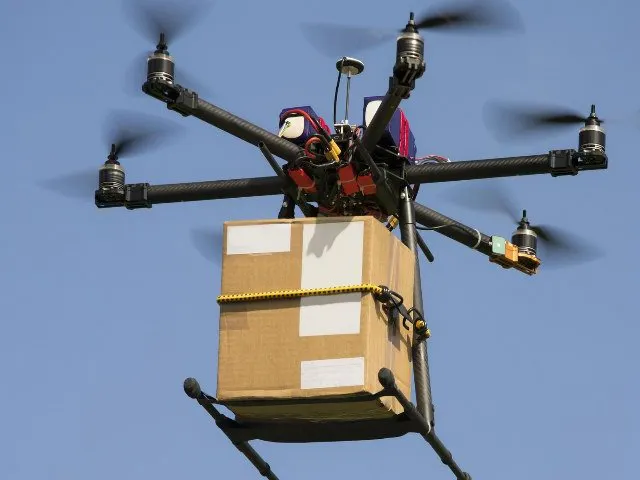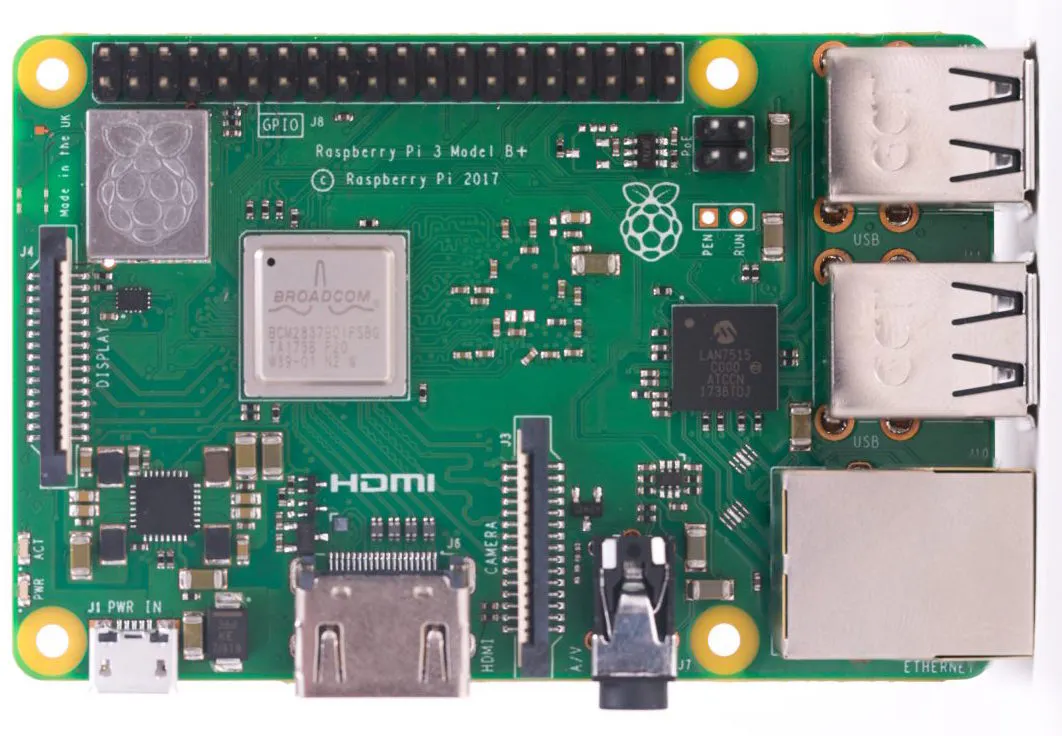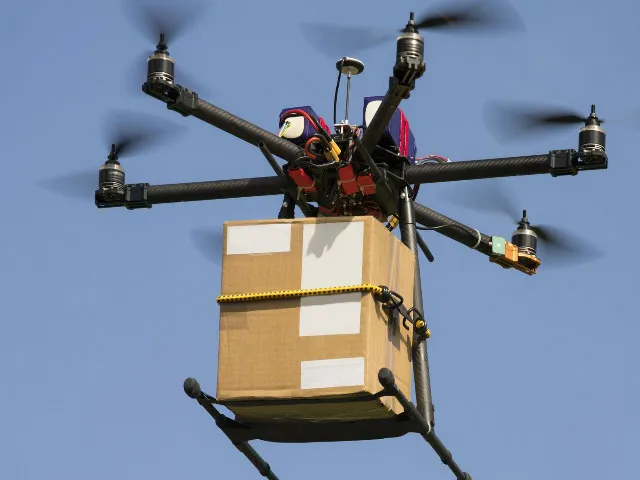# Most Clever Device in Industry Marketplace

Give your device the capability to choose the most profitable proposal request in Industry Marketplace.

IntermediateFull instructions providedOver 1 day582## Things used in this project

### Hardware componentsRaspberry Pi 3 Model B+
×2Flash Memory Card, SD Card
×1

### Software apps and online servicesRaspberry Pi RaspbianIOTA Tangle

## Schematics

### No schematic## Code

### The base class

Python
```import requests
import paho.mqtt.client as mqtt
import datetime
import json
import os
import pprint

class IndustryMarketplace:
'''
Abstract Industry Marketplace instance,
Should not be called directly but you should inherit it
with your own implementation class. See `service_provider.py`
for an example
'''

# Generic Marketplace settings, overwrite these to your needs
service_provider = False
name = 'Anonymous'
fund_wallet = False
gps_coords = None

# MQTT settings, leave as is to work out of the box with the ServiceApp
mqtt_timeout = 60
mqtt_port = 1883
mqtt_id = None
mqtt_broker = 'test.mosquitto.org'

# The endpoint of the ServiceApp Node application
endpoint = 'http://localhost:4000'
eclass = None
operations = None

def __init__(self):
self.mqtt_client = mqtt.Client()
self.update_eclass_whitelist()

def update_eclass_whitelist(self):
'''
Function to load the eclass whitelist or create it from it's source
if it does not exist. Only these IRDI's and Attributes can be used
with the Industry Marketplace right now.
'''
if not os.path.exists('eclass.json'):
with open('eclass.json', 'wb') as fh:
resp = requests.get('https://raw.githubusercontent.com/iotaledger/industry_4.0_language/master/catalog/eClass.json')
fh.write(resp.content)
self.eclass = resp.json()
else:

if not os.path.exists('operations.json'):
with open('operations.json', 'wb') as fh:
resp = requests.get('https://raw.githubusercontent.com/iotaledger/industry_4.0_language/master/catalog/operations.json')
fh.write(resp.content)
self.operations = resp.json()
else:

def get_price(self, irdi, submodels):
'''
Get the price for a irdi from the submodels
'''
try:
return [x['value'] for x in submodels.values() if x['idShort'] == 'price']
except IndexError:
return None

def get_location(self, irdi, submodels):
'''
Get the service location for a irdi from the submodels
'''
try:
return [x['value'] for x in submodels.values() if x['idShort'] == 'location [lat, lng]']
except IndexError:
return None

def get_total_weight(self, irdi, submodels):
'''
Get the cell tower range for a irdi from the submodels
'''
try:
return [x['value'] for x in submodels.values() if x['idShort'] == 'total weight (freight) [kg]']
except IndexError:
return None

def get_starting_point(self, irdi, submodels):
'''
Get the cell tower frequency for a irdi from the submodels
'''
try:
return [x['value'] for x in submodels.values() if x['idShort'] == 'starting point [lat, lng]']
except IndexError:
return None

def get_destination(self, irdi, submodels):
'''
Get the cell tower frequency for a irdi from the submodels
'''
try:
return [x['value'] for x in submodels.values() if x['idShort'] == 'destination [lat, lng]']
except IndexError:
return None

def get_number_of_photo(self, irdi, submodels):
'''
Get the energy consumption of BTS for a irdi from the submodels
'''
try:
return [x['value'] for x in submodels.values() if x['idShort'] == 'number of photos that can be stored']
except IndexError:
return None

def get_reliability_duration(self, irdi, submodels):
'''
Get the cell tower frequency for a irdi from the submodels
'''
try:
return [x['value'] for x in submodels.values() if x['idShort'] == 'reliability duration [min]']
except IndexError:
return None

def get_target_location(self, irdi, submodels):
'''
Get the cell tower frequency for a irdi from the submodels
'''
try:
return [x['value'] for x in submodels.values() if x['idShort'] == 'target location [lat, lng]']
except IndexError:
return None

def get_autonomous(self, irdi, submodels):
'''
Get the cell tower frequency for a irdi from the submodels
'''
try:
return [x['value'] for x in submodels.values() if x['idShort'] == 'autonomous']
except IndexError:
return None

def get_max_persons(self, irdi, submodels):
'''
Get the cell tower frequency for a irdi from the submodels
'''
try:
return [x['value'] for x in submodels.values() if x['idShort'] == 'max. number of persons']
except IndexError:
return None

def get_duration(self, irdi, submodels):
'''
Get the cell tower frequency for a irdi from the submodels
'''
try:
return [x['value'] for x in submodels.values() if x['idShort'] == 'duration[min]']
except IndexError:
return None

def get_max_valocity(self, irdi, submodels):
'''
Get the cell tower frequency for a irdi from the submodels
'''
try:
return [x['value'] for x in submodels.values() if x['idShort'] == 'maximum velocity at rated value [km/h]']
except IndexError:
return None

'''
Get the cell tower frequency for a irdi from the submodels
'''
try:
return [x['value'] for x in submodels.values() if x['idShort'] == 'max. monitoring radius [m]']
except IndexError:
return None

def get_2_4_value(self, irdi, submodels):
'''
Get the cell tower frequency for a irdi from the submodels
'''
try:
return [x['value'] for x in submodels.values() if x['idShort'] == '2,4 GHz']
except IndexError:
return None

def get_5_value(self, irdi, submodels):
'''
Get the cell tower frequency for a irdi from the submodels
'''
try:
return [x['value'] for x in submodels.values() if x['idShort'] == '5 GHz']
except IndexError:
return None

def get_energy_consumption(self, irdi, submodels):
'''
Get the cell tower frequency for a irdi from the submodels
'''
try:
return [x['value'] for x in submodels.values() if x['idShort'] == 'energy consumption [kW/h]']
except IndexError:
return None
####################################################################################################

def config(self):
data = {
'name': self.name,
'role': 'SP' if self.service_provider else 'SR',
'gps': self.gps_coords or '',
'wallet': self.fund_wallet
}

config = self.api('config', data=data)
if config.get('success', False):
self.log('Now running as %s under the name "%s"' % ('Service Provider' if self.service_provider else 'Service Requester', self.name))

return config

def api(self, uri, data=None):
try:
if data:
resp = requests.post('%s/%s' % (self.endpoint, uri), json=data, timeout=15)
#self.log('POST body: %s' % json.dumps(data))
return resp.json()
else:
resp = requests.get('%s/%s' % (self.endpoint, uri), timeout=15)
return resp.json()
except requests.exceptions.ConnectionError as e:
self.log('Unable to connect to Industry Marketmanager ServiceApp; Make sure it is running on %s' % self.endpoint)
exit(1)

def user(self):
user = self.api('user')
#self.log(user)
return user

def cfp(self, irdi, location=None, start_at=None, end_at=None, values=None):
'''
Send a call for proposal as a service requester

irdi: ecl@ss irdi number for requested service/product, see https://www.eclasscontent.com
location: LAT,LON
start_at: Datetime object, if None defaults to now
end_at: Datetime object, if None defaults to 10 minutes from now
values: Dict with as keys the property ID's and as values the property values as defined by ecl@ss
'''

if self.service_provider:
raise ValueError('Only Service Requesters are allowed to call this method')

if not location:
location = ''

if not start_at:
start_at = datetime.datetime.now() + datetime.timedelta(minutes=10)

if not end_at:
end_at = start_at + datetime.timedelta(minutes=20)

start_ts = int(start_at.timestamp()) * 1000
end_ts = int(end_at.timestamp()) * 1000

user = self.user()

data = {
'messageType': 'callForProposal',
'irdi': irdi,
'userId': user.get('id'),
'location': location,
'startTimestamp': start_ts,
'endTimestamp': end_ts,
'creationDate': datetime.datetime.now().strftime('%d %B, %Y %I:%M %p'),
'submodelValues': values or {},
}

#pprint.pprint(data)
ret = self.api('cfp', data=data)
#self.log(ret)
return ret

def proposal(self, proposal_data, price_in_iota):
'''
Send a proposal as a response to a call for proposal
Takes the original message it replies to as the `proposal_data` argument
'''

if not self.service_provider:
raise ValueError('Only Service Providers are allowed to call this method')

user = self.user()

irdi = proposal_data['dataElements']['submodels']['identification']['id']

data = {
'messageType': 'proposal',
'irdi': irdi,
'userId': user.get('id'),
'price': int(price_in_iota),
'originalMessage': proposal_data
}

ret = self.api('proposal', data=data)
#self.log(ret)
#print(ret)
return ret

def accept_proposal(self, proposal_data):

if self.service_provider:
raise ValueError('Only Service Requesters are allowed to call this method')

user = self.user()

irdi = proposal_data['dataElements']['submodels']['identification']['id']
price = proposal_data['frame'].get('price')

data = {
'messageType': 'acceptProposal',
'price': price,
'irdi': irdi,
'userId': user.get('id'),
'originalMessage': proposal_data
}

ret = self.api('acceptProposal', data=data)
return ret

def inform_confirm(self, proposal_data):

if not self.service_provider:
raise ValueError('Only Service Providers are allowed to call this method')

user = self.user()

irdi = proposal_data['dataElements']['submodels']['identification']['id']
price = proposal_data['frame'].get('price')

data = {
'messageType': 'informConfirm',
'irdi': irdi,
'price': price,
'userId': user.get('id'),
'originalMessage': proposal_data
}

ret = self.api('informConfirm', data=data)
return ret

def inform_payment(self, proposal_data):

if self.service_provider:
raise ValueError('Only Service Requesters are allowed to call this method')

user = self.user()

irdi = proposal_data['dataElements']['submodels']['identification']['id']
price = proposal_data['frame'].get('price')

data = {
'messageType': 'informPayment',
'irdi': irdi,
'price': price,
'userId': user.get('id'),
'originalMessage': proposal_data
}

ret = self.api('informPayment', data=data)
return ret

def reject_proposal(self, proposal_data):
if self.service_provider:
raise ValueError('Only Service Requesters are allowed to call this method')

user = self.user()

irdi = proposal_data['dataElements']['submodels']['identification']['id']
price = 100 #proposal_data['frame'].get('price')

data = {
'messageType': 'rejectProposal',
'irdi': irdi,
'userId': user.get('id'),
'price': price,
'originalMessage': proposal_data
}

ret = self.api('rejectProposal', data=data)
return ret

def listen(self, mqtt=False):
self.config()

data = self.api('mqtt', {'message': 'subscribe'})
if not data.get('success'):
raise ValueError('Unable to subscribe to MQTT stream: %s' % data.get('error'))

self.mqtt_id = data.get('id')
self.mqtt_client.on_connect = self.on_connect
self.mqtt_client.on_disconnect = self.on_disconnect
self.mqtt_client.on_message = self.on_message
self.mqtt_client.connect(self.mqtt_broker, self.mqtt_port, self.mqtt_timeout)
self.mqtt_client.loop_forever()

def log(self, message):
print('[%s] %s' % (datetime.datetime.now(), message))

def on_proposal(self, data, irdi=None, submodels=None):
'''
This function is called as soon as a proposal is received
Implement this function to do what you need as you please
'''

self.log('`[WARNING] on_proposal` function not implemented yet, you might want to implement this!')

def on_inform_confirm(self, data, irdi=None, submodels=None):
'''
This function is called as soon as a fulfillment has been confirmed
Implement this function to do what you need as you please
'''

self.log('`[WARNING] on_inform_confirm` function not implemented yet, you might want to implement this!')

def on_inform_payment(self, data, irdi=None, submodels=None):
'''
This function is called as soon as a payment has been completed
Implement this function to do what you need as you please
'''

self.log('`[WARNING] on_inform_payment` function not implemented yet, you might want to implement this!')

def on_cfp(self, data, irdi=None, submodels=None):
'''
This function is called as soon as a call for proposal is received
Implement this function to do what you need as you please
'''

self.log('`[WARNING] on_cfp` function not implemented yet, you might want to implement this!')

def on_accept_proposal(self, data, irdi=None, submodels=None):
'''
This function is called as soon as a proposal is accepted
Implement this function to do what you need as you please
'''

self.log('`[WARNING] on_accept_proposal` function not implemented yet, you might want to implement this!')

def on_reject_proposal(self, data, irdi=None, submodels=None):
'''
This function is called as soon as a proposal is rejected
Implement this function to do what you need as you please
'''

self.log('`[WARNING] on_reject_proposal` function not implemented yet, you might want to implement this!')

#TODO: Implement other callback functions

def on_message(self, client, userdata, message):
try:
except json.decoder.JSONDecodeError:
self.log('Unable to decode, no json found')

try:

try:
dat = data['data']['data']
first_request = dat['dataElements']['submodels']['identification']
irdi = first_request['id']
submodels = first_request['submodelElements']
submodeldict = dict([(x['semanticId'], x) for x in submodels])
except Exception as e:
self.log('Error getting data: %s' % e)

if data['data']['messageType'] == 'proposal':
self.on_proposal(dat, irdi=irdi, submodels=submodeldict)

elif data['data']['messageType'] == 'acceptProposal':
self.on_accept_proposal(dat, irdi=irdi, submodels=submodeldict)

elif data['data']['messageType'] == 'rejectProposal':
self.on_reject_proposal(dat, irdi=irdi, submodels=submodeldict)

elif data['data']['messageType'] == 'informConfirm':
self.on_inform_confirm(dat, irdi=irdi, submodels=submodeldict)

elif data['data']['messageType'] == 'informPayment':
self.on_inform_payment(dat, irdi=irdi, submodels=submodeldict)

elif data['data']['messageType'] == 'callForProposal':
self.on_cfp(dat, irdi=irdi, submodels=submodeldict)

else:
self.log('Unhandled message type: %s' % data['data']['messageType'])

except KeyError as e:
self.log('Invalid message format for data (%s) - %s' % (data, e))

def on_connect(self, client, *args, **kwargs):
self.log('connected')
self.mqtt_client.subscribe(self.mqtt_id)
self.log('subscribed to %s' % self.mqtt_id)

def on_disconnect(self, client, *arg, **kwargs):
self.log('mqtt disconnected')
```

### Service Provider

Python
```from imp import IndustryMarketplace
import random
from math import sin, cos, sqrt, atan2
import json
import pprint
proposal_receiving_start = False

class ServiceProvider(IndustryMarketplace):
name = 'RF Communication'
service_provider = True
fund_wallet = False
gps_coords = '23.123, 90.321'
proposed_price = random.randint(10, 20)
endpoint = 'http://192.168.0.103:4001'
R = 6373.0
irdi_list = []
profit_list = []
price_list = []

def sent_proposal(self):
"""This function is used to identify the most profitable request for the multiple requesters
and send the proposal to that request only."""
index_max = self.profit_list.index(max(self.profit_list))
proposed_irdi = self.irdi_list[index_max]
proposed_price = int(self.price_list[index_max])
with open(proposed_irdi + '.txt') as json_file:
try:
ret = self.proposal(data, price_in_iota = proposed_price)
except Exception as e:
self.log('Unable to send proposal', e)

self.log('proposal sent! Requesting %si for this service' % self.proposed_price)

def on_cfp(self, data, irdi, submodels):
'''
As soon as this service provider receives a CfP this function is called
You can read the requester's requirements from submodels to define a price if you like
'''

self.log('Call for Proposal received for irdi %s!' % irdi)

if irdi == '0173-1#01-AAJ336#002':   #irdi for drone transport service
try:
"""Reads the irdi attributes from the call for proposal and check the capability and calculate price."""
starting_point = self.get_starting_point(irdi, submodels)
destination = self.get_destination(irdi, submodels)
total_weight = self.get_total_weight(irdi, submodels)
"""This portion is used to check the capability of providing service mentioned to the call for proposal
and return if does not meet."""
if total_weight>5:
return   #assuming it only can carry 5 Kg
"""This portion is used to write the received data and written to a text file with irdi number so
that it can be used when sending proposal."""
with open(irdi + '.txt', 'w') as json_file:
json.dump(data, json_file)
"""After getting starting point and destination I am calculating distance and from that distance
and weight I am calculating the price just using a random equation. I also calculating the profit
assuming 35% of the total price."""
lat1 = starting_point.split(',')
lon1 = starting_point.split(',')
lat2 = destination.split(',')
lon2 = destination.split(',')
dlon = float(lon2) - float(lon1)
dlat = float(lat2) - float(lat1)
a = (sin(float(dlat) / 2)) ** 2 + cos(float(lat1)) * cos(float(lat2)) * (sin(float(dlon) / 2)) ** 2
c = 2 * atan2(sqrt(a), sqrt(1 - a))
distance = self.R * c
price = distance * 1.2 + total_weight * 1.2
self.log('price')
self.log(price)
profit = 0.35 * price
self.irdi_list.append(irdi)
self.price_list.append(price)
self.profit_list.append(profit)

print('Received proposal request to carry = %s Kg for %s Km, for irdi %s' % (
total_weight, distance, irdi))
"""Wait for three proposal requests before sending the proposal to determine most profitable one."""
if len(self.irdi_list) >= 3:
self.sent_proposal()

except Exception as e:
self.log('Error on accepting: %s' % e)

elif irdi == '0173-1#01-AAP788#001':   #irdi for drone inspection service
try:
duration = self.get_reliability_duration(irdi, submodels)
location = self.get_location(irdi, submodels)
number_of_photo = self.get_number_of_photo(irdi, submodels)
self.log('step 21')
self.log(duration)
self.log(number_of_photo)
if duration > 20 or number_of_photo > 50:
return
"""I am assuming that the price depends on duration and number of photos. So againg I am using a random
equation to calculate price and profit for demonstration purpose only."""
with open(irdi + '.txt', 'w') as json_file:
json.dump(data, json_file)
self.log('step 23')
price = 7 + duration*1.2 + number_of_photo
profit = 0.42 * price
self.irdi_list.append(irdi)
self.price_list.append(price)
self.profit_list.append(profit)
print('Received proposal request for inspection  for  %s minutes, %s photos'
'for location %s for irdi %s' % (
duration, number_of_photo, location, irdi))

if len(self.irdi_list) >= 3:
self.sent_proposal()

except Exception as e:
self.log('Error on accepting: %s' % e)

elif irdi == '0173-1#01-AAI711#001':  # irdi for mobility service
try:
starting_point = self.get_starting_point(irdi, submodels)
destination = self.get_destination(irdi, submodels)
max_person = self.get_max_persons(irdi, submodels)
autonomous = self.get_autonomous(irdi, submodels)
"""I am assuming that the price depends on all the parameters. So again I am using a random
equation to calculate price and profit for demonstration purpose only."""
with open(irdi + '.txt', 'w') as json_file:
json.dump(data, json_file)
lat1 = starting_point.split(',')
lon1 = starting_point.split(',')
lat2 = destination.split(',')
lon2 = destination.split(',')
dlon = float(lon2) - float(lon1)
dlat = float(lat2) - float(lat1)
a = (sin(float(dlat) / 2)) ** 2 + cos(float(lat1)) * cos(float(lat2)) * (sin(float(dlon) / 2)) ** 2
c = 2 * atan2(sqrt(a), sqrt(1 - a))
distance = self.R * c
if distance > 900 or max_person > 45:
return
if autonomous:
price = 5 + distance * 1.2 + max_person
else:
price = 2 + distance * 1.1 + max_person
profit = price * 0.35
self.irdi_list.append(irdi)
self.price_list.append(price)
self.profit_list.append(profit)
print('Received proposal request for mobility service from %s to %s'
'to carry % person, for irdi %s' % (
starting_point, destination, max_person, irdi))

if len(self.irdi_list) >= 3:
self.sent_proposal()

except Exception as e:
self.log('Error on accepting: %s' % e)

else:
self.log('The work is out of our scope!')
return

def on_accept_proposal(self, data, irdi, submodels):
self.log('Proposal accepted! Start fulfilling')
self.log('Sending inform confirm')
ret = self.inform_confirm(data)
# self.log(ret)

def on_reject_proposal(self, data, irdi, submodels):
self.log('Proposal rejected!')
price = self.get_price(irdi, submodels)
marginal_price = 0.8*self.proposed_price
if price > marginal_price:
try:
ret = self.proposal(data, price_in_iota=price * 0.8)
self.log('20% Discount is offered!')
except Exception as e:
self.log('Unable to send proposal', e)
else:
self.log('Proposal rejected even after 20% discount!')

def on_inform_payment(self, data, irdi, submodels):
# pprint.pprint(data)

if __name__ == '__main__':
imp = ServiceProvider()
imp.listen()
```

### Service Requester

Python
```from imp import IndustryMarketplace
import pprint
import sys

class ServiceRequester(IndustryMarketplace):
name = 'MakerBangla'
service_provider = False
fund_wallet = True
gps_coords = '23.759, 90.378'

endpoint = 'http://192.168.0.102:4000'  # or your localhost

def on_proposal(self, data, irdi, submodels):
'''
Accept only for cell tower rent request
'''
self.log('on proposal called!')
try:
price = self.get_price(irdi, submodels)
self.log('Received proposal for %si for irdi %s' % (price, irdi))
if not price:

if price <= 50:
self.log('Accepting proposal')
self.accept_proposal(data)
else:
self.log('Rejecting proposal')
self.reject_proposal(data)
except Exception as e:
self.log('Error on accepting: %s' % e)

def on_inform_confirm(self, data, irdi, submodels):
self.log('Offer confirmed, time to pay')
self.inform_payment(data)

if __name__ == '__main__':

imp = ServiceRequester()
imp.listen()

```

## Credits

### Md. Khairul Alam

55 projects • 521 followers
Developer, Maker & Hardware Hacker. Currently working as a faculty at the University of Asia Pacific, Dhaka, Bangladesh.

### Umme Nur Mafiha Majid

1 project • 5 followers
I love technology